#### Get Our New Book!A loop is any path through a circuit that starts and ends at the same point. Consider, for example, the Ampeg B-22-X high-gain input circuit. Here are three possible loops: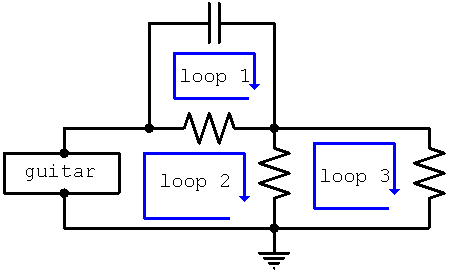These are not all the loops in the circuit. For example the path from ground through the guitar, through the capacitor, through the right-most resistor, and back to ground is also a loop.

#### Gustav Robert Kirchhoff's Voltage Law

According to Kirchhoff's Voltage Law, or KVL as it is known, the sum of the voltage rises around a closed loop equals the sum of the voltage drops.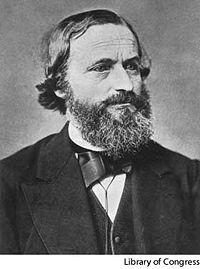#### Problem

Show that Kirchhoff's Voltage Law applies to one of the ripple filters in a Traynor YBA-3.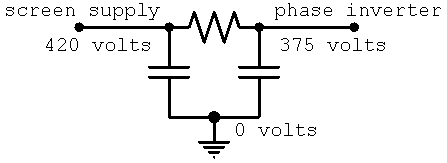#### Solution

There is only one closed loop shown. Starting at the screen supply and working clockwise, there is a voltage drop of 45 volts to 375 volts. Then there is a voltage drop of 375 volts to zero volts. Finally there is a voltage rise of 420 volts to 420 volts. Thus there is only one voltage rise and it equals the sum of the voltage drops:

420 = 45+375

For any other starting point, clockwise or counterclockwise, KVL would also hold.

#### KVL in Practice

Kirchhoff's Voltage Law is used often in circuit analysis because it allows us to determine an unknown voltage based on known voltages. The choice of which loop to use is a matter of convenience.

#### Problem

Given that the cathode of an Ampeg B-42-X preamp tube is at 2.5 volts with respect to ground, what is the grid-to-plate voltage? The grid is at ground potential (0 volts).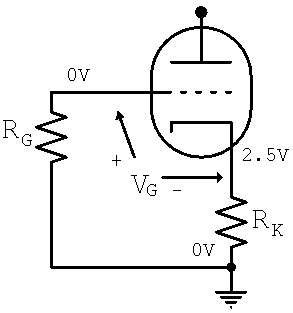#### Solution

We can form a counterclockwise loop from the grid, through the grid resistor RG to ground, through the cathode resistor RK to the cathode, and finally from the cathode to the grid, where we have indicated that we expect a rise of V volts. If this expectation turns out to be incorrect, the value of V will simply be negative. In this loop there are two voltage rises, V from cathode to grid and 2.5 volts from ground to cathode. There are no voltage drops. According to Kirchhoff's Voltage Law

VG+2.5 = 0

This means

VG = -2.5

The grid voltage, by which we mean the voltage of the grid relative to the cathode, is thus -2.5 volts.

This was a very simple loop and we could easily have determined the answer just by looking at the circuit. Try doing this with a Fender Bassman 5F6-A or Hiwatt DR103 tone stack! KVL provides us a formal, step-by-step procedure to keep from making mistakes, no matter how complicated the circuit gets.

#### Problem

Your Princeton 5F2 clone uses an output transformer that is, like all transformers, far from perfect. For DC voltages its resistance should ideally be zero, but using your vintage Hewlett Packard Model 410B vacuum tube voltmeter you measure an internal winding resistance of 50 ohms. The plate supply voltage is 305 volts and the voltage across the 470-ohm cathode resistor is 19 volts. What is the plate current that flows from the 305-volt supply, through the transformer, through the tube, and finally through the cathode resistor? What is the 6V6GT plate voltage, measured from plate to cathode?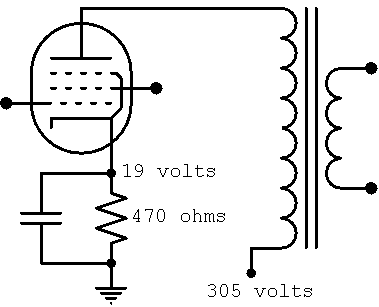Guitar Amplifier Electronics: Basic Theory - a modern approach to understanding tube amp design. Learn more.Guitar Amplifier Preamps - upstream circuits using triodes and pentodes. Learn more.Guitar Amplifier Power Amps - from the phase inverter to the loudspeaker. Learn more.The Fender Bassman 5F6-A - plus Marshall's Bluesbreaker and Plexi. Learn more.Georg Simon Ohm - Imagine a world without Ohm's Law. Learn more.